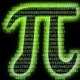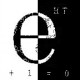奇异值分解和图像压缩

【2.18更新】：楠神写了一个非常gelivable的Shiny应用，用来动态展示图片压缩的效果随k的变化情况。谢大大把这个应用放到了RStudio的服务器上，大家可以点进去玩玩看了。

=====================代表正义的分割线=====================

$$M=UDV^T$$

• $U$ 是一个 $m\times n$ 的矩阵，满足 $U^T U=I_n$，$I_n$ 是 $n\times n$ 的单位阵
• $V$ 是一个 $n\times n$ 的矩阵，满足 $V^T V=I_n$
• $D$ 是一个 $n\times n$ 的对角矩阵，所有的元素都非负

$$U=(u_1,u_2,\ldots,u_n)$$

$$V=(v_1,v_2,\ldots,v_n)$$

$$M=d_1u_1v_1^T+d_2u_2v_2^T+\cdots+d_nu_nv_n^T=\sum_{i=1}^n d_iu_iv_i^T=\sum_{i=1}^n A_i$$

$$M_k=\sum_{i=1}^k A_i$$

【注：本文只是为了用图像压缩来介绍 SVD 的性质，实际使用中常见的图片格式（png，jpeg等）其压缩原理更复杂，且效果往往更好】

library(rARPACK);
library(jpeg);

factorize = function(m, k)
{
r = svds(m[, , 1], k);
g = svds(m[, , 2], k);
b = svds(m[, , 3], k);
return(list(r = r, g = g, b = b));
}

recoverimg = function(lst, k)
{
recover0 = function(fac, k)
{
dmat = diag(k);
diag(dmat) = fac$d[1:k]; m = fac$u[, 1:k] %*% dmat %*% t(fac$v[, 1:k]); m[m < 0] = 0; m[m > 1] = 1; return(m); } r = recover0(lst$r, k);
g = recover0(lst$g, k); b = recover0(lst$b, k);
m = array(0, c(nrow(r), ncol(r), 3));
m[, , 1] = r;
m[, , 2] = g;
m[, , 3] = b;
return(m);
}

lst = factorize(rawimg, 100);
neig = c(1, 5, 20, 50, 100);
for(i in neig)
{
m = recoverimg(lst, i);
writeJPEG(m, sprintf("svd_%d.jpg", i), 0.95);
}《奇异值分解和图像压缩》有18个想法

1.酱油小厮说道：

牛逼！

2.road2stat说道：

火钳流明！哈哈，SVD 就是 PCA，PCA 就是 MDS 。。。

可以配着小曲儿读。It Had To Be U: The SVD Song
http://v.youku.com/v_show/id_XNDkxMTgzNzU2.html

1.linkinbird说道：

我们一直生活在一个超高维度的空间，只是人类的肉眼太弱了……哈哈哈哈

1.road2stat说道：

通过人类聪明的大脑可以脑补 。。。

3.布丁Nnn说道：

小轩哥出品必属精品啊，忍不住过来怒赞！！！ 不如写一个系列吧，SVD的前世今生～～

4.yangzhixuan说道：

不该把U定义成m乘n的，别人本来是R^m的标准正交基，原矩阵的行空间和零空间的基底，你平白无故给他切掉了几列，毫无必要，还假设了m比n大

1.Yixuan说道：

同意你说的，但这里用的是所谓的窄版SVD，因为从矩阵近似的角度来说，剩下的几组基底是没有用的。m>n的问题好办，转置一下再分解就是。主要是不想涉及太多概念上的东西，就把一些内容省掉了。

5.Bing说道：

好文，赞一个。
"SVD 和特征值分解有着非常紧密的联系，此为后话"。 这个后话也值得详细写一篇：U和V的得来以及各自如何解释才是SVD更加引人入胜的地方：归根结底还是谱分解定理的扩展，是PCA在行空间和列空间的推广。

6.严酷的魔王说道：

要是能列出k=1~5的图层分别是什么样的就好了，还有d1~d5的数值，可以直观地看出数值的贡献~

7.谢益辉说道：

帅气！谁来搞个Shiny app，用滑动条来选择k，实时看结果？

1.Yixuan说道：

计算量还是有点大的，除非把图片预先生成好，不过那就是放幻灯片了，哈哈。

8.诺敏说道：

好文，学习了

9.sglidq说道：

SVD的文章，赞一个！

10.Sean说道：

SVD 和特征值分解有着非常紧密的联系，此为后话
U和V的得来以及各自如何解释才是SVD更加引人入胜的地方：归根结底还是谱分解定理的扩展，是PCA在行空间和列空间的推广

期待后话！！

11.般若紫丁说道：

学长你好，我想请教一下rainbow软件包的使用问题：软件包里示例数据我看到都是月度数据，我想用该包来展示年度数据的相关图形，结果怎么也运行不出来。
比如：foutliers(scicbj, method = "depth.trim")
错误于apply(functions[lista, ], 2, mean) :
dim(X) must have a positive length
这里scicbj的结构和示例中的ElNino是相同的，只不过是scicbj中x为1，示例为12（例子是月度数据）。
这个包是本来就不能处理年度数据吗？还是我哪里出了问题呢？

1.Yixuan说道：

这个与之前一条评论相关，维基中给出的是完整版的SVD，我这里用的是紧凑版的。